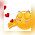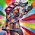Stella Dimoko Korkus.com: Number Puzzle.......

## Friday, April 03, 2020

### Number Puzzle.......

Who can solve this????

1.2.1+3+5 equals to 9.
Purported number(18)-9 equals to 9.

3.This one na quantitative reasoning.
Is it no.4?🙄
Over to Teacher Nkay, Rhoda, Tynu Akom, Mercy et al🤔

4.Abeg no idea. I suck at quantitative reasoning 😌

1.5.6.7.1.8.9.3. I think it's 1 + 2= 3.

10.9+1=10
6+1=7
10-7= 3

2+9=11
3+6=9
11-9=2

7+3=10
6+3=9
10-9=1

1+5=6
3+3=6
6-6=0

From the answers above, they filled numerically 3,2,1, 0

So the missing answer is 3

1.I agree with this, this was my first answer, but anon 9:10 has a valid sequence as well. I guess there are 2 ways to look at it.

11.I think the correct answer is 9.

12.13.14.1 2 3 4 5 6 7 8 9

Choose anyone. 😁

1.Heheheheheheheh

15.7+3=10
6+3=9
10-9=1

1+9=10
5+3=8
10-8=2

9+1=10
6+1=7
10-7=3

9+3=12
2+6=8
12-8=4

16.17.The first is a total of 17, the second needs 9 to be 18, the third is 19, the last is a total of 20.

18.Or it could be 9
2+3+6+9=20
7+3+6+3=19
3+1+5+x=18
9+6+1+1=17
So x may be=18-9=9

19.I no fit jor

20.The answer is 9. The first square total is 20, the second is 19, the fourth square is 17 to get the total of 18 in the third square, you add 9. My son solved it..

21.Stella,thank you for the free data that I just received. Beloved,I thank you for picking me too.God bless you both.If I remember,I'd try to post my thanks again in any of the post on the blog,tomorrow.Thanks.

Stella,pls post this.

22.Disclaimer: Comments And Opinions On Any Part Of This Website Are Opinions Of The Blog Commenters Or Anonymous Persons And They Do Not Represent The Opinion Of StellaDimokoKorkus.com

Pictures and culled stories posted on this site are given credit and if a story is yours but credited to the wrong source,Please contact Stelladimokokorkus.com and corrections will be made..